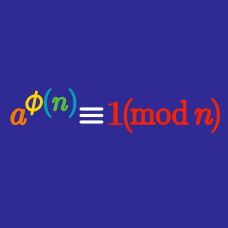Number Theory

Euler's Theorem: Level 5 Challenges

$\Large 9^{8^{7^{6^{5^{4^{3^{2^{1}}}}}}}}$

Find the last three digits of the above number.

Let $a$ and $b$ be positive integers with $a>b$ such that $7!\Big|\big(x^a-x^b\big)$ for all integers $x.$

Find the smallest possible value of $a+b.$

Clarification: $!$ denotes the factorial notation. For example, $8! = 1\times2\times3\times\cdots\times8$.

$\large 2,2^2,2^{2^2},2^{2^{2^2}},\ldots$ The sequence above can be recursively defined by $a_1=2, a_{k+1}=2^{a_{k}}$

Given a positive integer $n$, a new sequence is created by dividing each term $\{a_k\}$ by $n$ and writing down the remainder of the division. We denote this new sequence by $\{b_{k}\}$.

For a given $n$, it is known that the terms of $\{b_{k}\}$ eventually become constant. Let $f(n)$ denote the index at which the constant values begin, i.e. $f(n)$ is the smallest number $i$ for which $b_{i-1} \neq b_i = b_{i+1} = b_{i+2} = \cdots$.

Find $f(2016)+f(2015)$.

Proving that the terms of $\{b_{n}\}$ become constant is an old USAMO problem, which inspired this problem.

How many integers $1\leq{a}\leq{2015}$ are there such that $a^{a^a}$ and $a^a$ end with the same digit?

$\Large 249^3 = 15438\underline{249}$

An automorphic number is defined as a positive integer $n$ such that the trailing digits of $n^m$, where $m$ is a positive integer, is $n$ itself for all $m>0$.

Let us define an almost automorphic number as a number where $n$ only appears as the trailing digits of $n^m$ for all odd $m>0$. How many almost automorphic numbers are less than 1000?

×